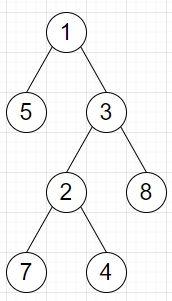# Program to find difference between node and a descendent in Python

Suppose we have a binary tree, we have to find the largest absolute difference between any node and its descendants.

So, if the input is likethen the output will be 7 as largest absolute difference is between nodes 8 and 1.

To solve this, we will follow these steps −

• Define a function dfs() . This will take node
• if node is not null, then
• return a list with positive and negative infinity
• left := dfs(left of node)
• right := dfs(right of node)
• res := a pair with (minimum of left, right and value of node, and maximum of left, right and value of node)
• ans := maximum of ans, (value of node - res) and (res - value of node)
• return res
• From the main method, do the following −
• ans := 0
• dfs(root)
• return ans

Let us see the following implementation to get better understanding −

## Example

Live Demo

class TreeNode:
def __init__(self, data, left = None, right = None):
self.val = data
self.left = left
self.right = right
class Solution:
def solve(self, root):
def dfs(node):
if not node:
return [float("inf"), float("-inf")]
left = dfs(node.left)
right = dfs(node.right)
res = [min(left, right, node.val), max(left, right, node.val)]
self.ans = max(self.ans, node.val - res, res - node.val)
return res
self.ans = 0
dfs(root)
return self.ans
ob = Solution()
root = TreeNode(1)
root.left = TreeNode(5)
root.right = TreeNode(3)
root.right.left = TreeNode(2)
root.right.right = TreeNode(8)
root.right.left.left = TreeNode(7)
root.right.left.right = TreeNode(4)
print(ob.solve(root))

## Input

root = TreeNode(1)
root.left = TreeNode(5)
root.right = TreeNode(3)
root.right.left = TreeNode(2)
root.right.right = TreeNode(8)
root.right.left.left = TreeNode(7)
root.right.left.right = TreeNode(4)

## Output

7